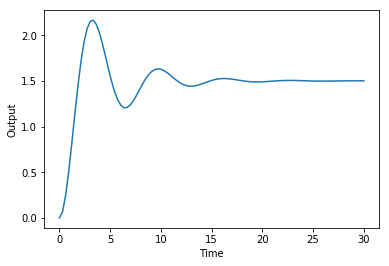# Simulating a plant with time-dependent control actions¶

Todo: clean up descriptions and libraries

Assume that we have a plant with some simple dynamics described by this transfer function:

$$G(s) = \frac{1}{s^2 + 2s + 1}$$

We can define the system in python-control by doing:

In :
import control
import numpy as np
import matplotlib.pyplot as plt
%matplotlib inline

G_s = control.tf([1.5],[1,0.5,1])
G_s

Out:
      1.5
---------------
s^2 + 0.5 s + 1

### Step Response: control.step_response¶

Here's how to get the step response and plot the output:

In :
# Generate a step response
T = np.linspace(0,30,100)
t, yout = control.step_response(G_s,T)

# Plot
plt.figure()
plt.xlabel('Time')
plt.ylabel('Output')
plt.plot(t,yout)

Out:
[<matplotlib.lines.Line2D at 0x11d697d68>]## Output with control actions: control.forced_response¶

What if we want to introduce the step at time $t=20$s? We can do this by using control.forced_response and feeding in an input vector, $u$ containing the control actions at each time step:

In :
# Generate a step response
T = np.linspace(0,100,1000)

# Our input is all zero, until t=20
U = np.ones(len(T))
U[:200] = 0

t, yout, _ = control.forced_response(G_s, T, U)

# Plot
plt.figure()
plt.xlabel('Time')
plt.ylabel('Output')
plt.plot(t,yout)
plt.step(t,U, linestyle='--') # We'll use step to create a staircase plot for the control actions

Out:
[<matplotlib.lines.Line2D at 0x11db15940>]

Here's the response with 2 control actions, one at $t=20$s and another one at $t=60$s:

In :
# Generate a step response
T = np.linspace(0,100,1000)

# Our input is one between t=20 and t=60
U = np.zeros(len(T))
U[200:600] = 1

t, yout, _ = control.forced_response(G_s, T, U)

# Plot
plt.figure()
plt.xlabel('Time')
plt.ylabel('Output')
plt.plot(t,yout)
plt.step(t,U, linestyle='--')

Out:
[<matplotlib.lines.Line2D at 0x11e2085c0>]

## Animated outputs¶

Let's create some animations.

In :
# Start by importing our animation libraries
%config InlineBackend.figure_formats = {'svg',}
import matplotlib.pylab as plt
import matplotlib.gridspec as gridspec
from IPython.display import display
from matplotlib import animation
from IPython.display import HTML

In :
# Generate a step response
T = np.linspace(0,20,100)

# Our input is one between t=15 and t=30
U = np.zeros(len(T))
U[10:50] = 1

# TF
G_s = control.tf([1.0],[0.5,0.5,1])

t, yout, _ = control.forced_response(G_s, T, U)

In :
G_s

Out:
         1
-------------------
0.5 s^2 + 0.5 s + 1
In :
# Create sub plots
gs = gridspec.GridSpec(2,1)
f = plt.figure(figsize=(8,6));
ax1 = plt.subplot(gs[0, 0]); # row 0, col 0
ax2 = plt.subplot(gs[1, 0],sharex=ax1); # row 1, col 0
plt.setp(ax1.get_xticklabels(), visible=False)
f.set_tight_layout('tight')
line1, = ax1.plot(t, yout, lw=1.5);
line2, = ax2.step(t, U, lw=1.5, linestyle='--', color='r');

ax1.set_ylabel('Output')
ax2.set_ylabel('Action')
ax2.set_xlabel('Time (s)')

# How many data points to skip for plotting
skip = 1

# The animation function. This is called sequentially, frame by frame, to create the animated plot.
def drawframe(n):
line1.set_data(t[0:n*skip:skip], yout[0:n*skip:skip])
line2.set_data(t[0:n*skip:skip], U[0:n*skip:skip])

# Print out the current frame using "\r", the 'carriage return' character, as our end character.
# This makes Python print the frame on the same line.
print("Frame: {:0d}".format(n), end="\r")
return (line1,line2)

In :
# The interval parameter is the delay between frames in milliseconds and it controls the speed of the animation.
anim = animation.FuncAnimation(f, drawframe, frames=round(len(t)/skip), interval=20)
HTML(anim.to_jshtml())

Frame: 99

Out:

Once Loop Reflect